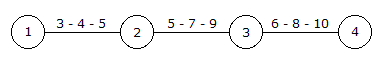# Civil Engineering - Construction Management

### Exercise :: Construction Management - Section 2

26.

Pick up the correct statement from the following:

 A. Forward pass is used for calculating earliest expected time B. Backward pass is used for calculating the latest occurence time C. Maximum value of earliest expected time is used if there are more than one value of any event D. Minimum value of latest occurrence time is used if there are more than one value of any event E. All the above.

Explanation:

No answer description available for this question. Let us discuss.

27.

Which one of the following represents an event ?

 A. concrete cured B. fixing of door C. plastering of walls D. selecting sites E. all the above.

Explanation:

No answer description available for this question. Let us discuss.

28.

Pick up the incorrect statement from the following:

 A. The various functions under each activity, are shown by one bar on Bar Charts B. Bar chart establishes the interdependency of one event on another C. Only approximate percentage of the completed work is reported D. None of these,

Explanation:

No answer description available for this question. Let us discuss.

29.

For the network shown in the given figure, the expected time for the activityA. 1-2 is 4 B. 2-3 is 7 C. 3-4 is 8 D. all the above.

Explanation:

Expected time = (Pessimistic value + 4*most likely value + Optimistic value)/6.

1-2 is 4.

2-3 is 7.

3-4 is 8.

30.

Frequency distribution curves

 A. having a single lump, are called uninodal curves B. if symmetrical, are called normal curves C. if not symmetrical, are called skew curves D. all the above.